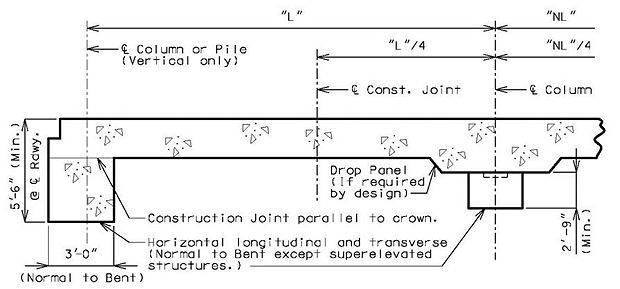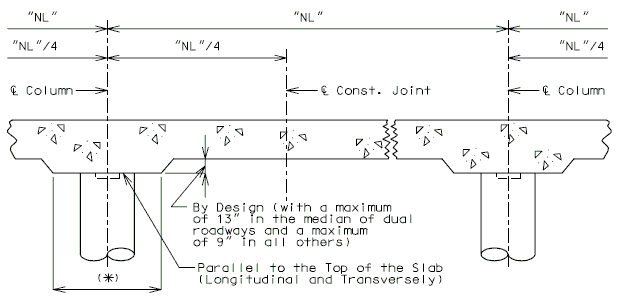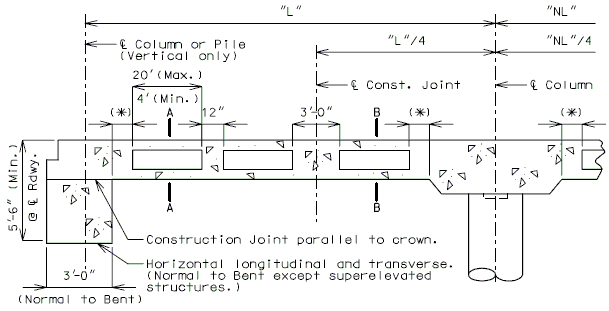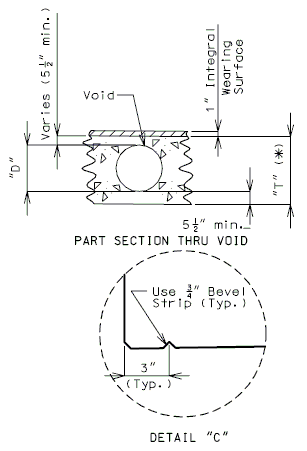# 751.17 Concrete Slab Bridges

## 751.17.1 General

This article illustrates the general design procedure for Continuous Concrete Slab Bridge using AASHTO LRFD Bridge Design Specifications.

### 751.17.1.1 Material Properties

Concrete:
Unit weight of reinforced concrete, $\,{\boldsymbol {\gamma }}_{c}=0.150kcf$Continuous Cast-In-Place Solid/Voided Concrete Slab
Class B-2 $\,f'_{c}=4.0ksi$$\,n=8$Precast Prestressed Multicell Voided Concrete Girders
Class A-1 $\,f'_{c}=6.0ksi$$\,f'_{ci}=4.5ksi$<math\, >n=8[/itex]
Intermediate bent columns, end bents (below construction joint at bottom of slab)

in continuous concrete slab bridges

Class B-1 $\,f'_{c}=4.0ksi$$\,n=8$Class B, Open bent, footing $\,f'_{c}=3.0ksi$$\,n=10$Modulus of elasticity,

$E_{c}=33,000\ K_{1}\ (w_{c}^{1.5}){\sqrt {f_{c}^{'}}}$Where:
f'c in ksi
wc = unit weight of nonreinforced concrete = 0.145 kcf
K1 = correction factor for source of aggregate
---- = 1.0 unless determined by physical testing
Modulus of rupture,

$\,f_{r}$= 0.24 $\,{\sqrt {f_{c}^{'}}}$LRFD 5.4.2.6

Where:
f'c in ksi

Reinforcing steel:
Minimum yield strength, $\,f_{y}=60.0ksi$Steel modulus of elasticity $\,E_{s}=29000ksi$Unit weight of future wearing surface, $\,{\boldsymbol {\gamma }}_{fws}=140lb./ft^{3}$## 751.17.2 Design

### 751.17.2.1 Limit States and Load Factors

In general, each component shall satisfy the following equation:

$\,Q=\textstyle \sum \eta _{i}\gamma _{i}Q_{i}\leq \phi R_{n}=R_{r}$Where:

 $\,Q$= Total factored force effect $\,Q_{i}$= Force effect $\,\eta _{i}$= Load modifier $\,\gamma _{i}$= Load factor $\,\phi$= Resistance factor $\,R_{n}$= Nominal resistance $\,R_{r}$= Factored resistance

Limit States

The following limit states shall be considered for slab and edge beam design:

STRENGTH - I
SERVICE - I
FATIGUE
EXTREME EVENT - II

Resistance factors

For STRENGTH limit state,

Flexure and tension of reinforced concrete, $\phi$= 0.90
Shear and torsion, $\,\phi$= 0.90

For all other limit states, $\,\phi$= 1.00

Slab weight
Future Wearing Surface
A 3” thick future wearing surface (35psf) shall be considered on the roadway.
Barrier/Railing
For slab overhang design, assume the weight of the barrier or railing acts at the centroid of the barrier or railing.
 * 2'-0" Min. ** 12" For deck overhang design (LRFD 3.6.1.3.1), 2’-0" for design of all other components
Application of Live Load to Slab

Vehicular
The design vehicular live load HL-93 shall be used. It consists of either the design truck or a combination of design truck and design lane load.
For slab design, where the primary strips are longitudinal, the force effects shall be determined on the following basis:
• The longitudinal strips shall be designed for all loads specified in AASHTO Article 3.6.1.3.3 includuing lane load.
• For the purpose of slab design, the lane load consists of a load equal to 0.640 klf uniformly distributed over 10 feet in the transverse direction.

For precast prestressed multicell girders, live load shall be distributed according to AASHTO LRFD Tables 4.6.2.2.2b-1, 4.6.2.2.2d-1, 4.6.2.2.3a-1 and 4.6.2.2.3b-1 for both moment and shear.

The dynamic load allowance replaces the effect of impact used in AASHTO Standard Specifications. It accounts for wheel load impact from moving vehicles. For slabs, the static effect of the vehicle live load shall be increased by the percentage specified in Table below.

Dynamic Load Allowance, $\,IM$Slab Component $\,IM$Deck Joints – All Limit States 75%
All Other Limit States 33%

The factor to be applied to the static load shall be taken as:

$\,(1+IM)$The dynamic load allowance is not to be applied to pedestrian or design lane loads.

Multiple Presence Factor, $\,m$:

The multiple presence factor accounts for the probability for multiple trucks passing over a multilane bridge simultaneously.

 $\,m$= 1.20 for 1 Loaded Lane 1.00 for 2 Loaded Lanes 0.85 for 3 Loaded Lanes 0.65 for more than 3 Loaded Lanes

Pedestrian

Pedestrian live load on sidewalks greater than 2 ft wide shall be:

 $\,PL$= 0.075 ksf

This does not include bridges designed exclusively for pedestrians or bicycles.

## 751.17.3 Details

### 751.17.3.1 Solid Slabs

SLAB LONGITUDINAL SECTIONS - SOLID SLABSEND SPANSINTERMEDIATE SPANS

Note:
All longitudinal dimensions shown are horizontal.

### 751.17.3.2 C.I.P. Voided Slabs

SLAB LONGITUDINAL SECTIONS - CAST-IN-PLACE VOIDED SLABEND SPANSINTERMEDIATE SPANS
(*) 3'-0" or greater than or equal to 5% of span length.
(**) By Design (6" increments measured normal to the centerline of bent) (The minimum is
equal to the column diameter + 2'6")

Note:
All longitudinal dimensions shown are horizontal (Bridges on grades and vertical curves, included).
For sections A-A and B-B, see below.

SLAB CROSS SECTIONHALF SECTION A-A CENTER OF SPAN HALF SECTION B-B NEAR INTERMEDIATE BENT

 Sonovoids are produced in halfsizes 2" to 18". D = 4" to 36"  T=19" (Min. preferred. ConsultStructural Project Manager prior to the use of a thinner slab.)Notes:
(*)   Increase the Dimension "T" by 1/2" for #14 bars placed in the top or bottom of the slab.

Increase the Dimension "T" by 1" for #14 bars placed in the top and bottom of the slab.
("T" and "D" are based on 3" clearance which includes the integral wearing surface to the top of the longitudinal bar.)

(**)  For Roadway with slab drains, use 10" minimum. For Roadways that require additional reinforcement for resisting moment of the edge beam 20" minimum. Check for adequate space for development of barrier or railing reinforcement.